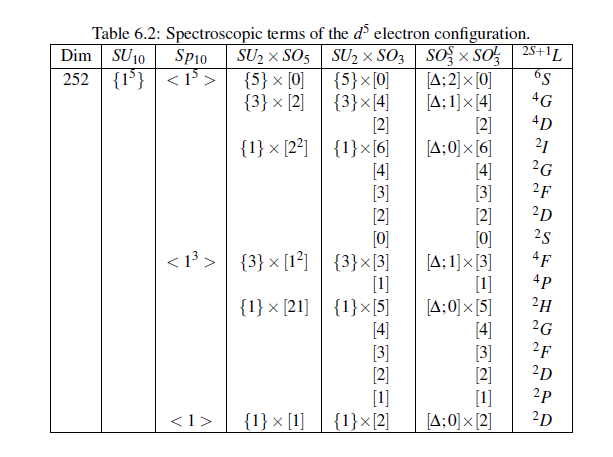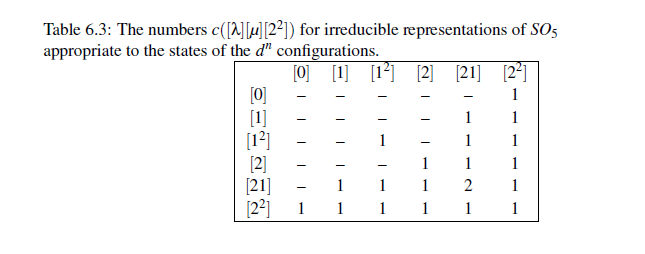# Schur マニュアルの問題を解く　その２

### 6-6節 Exercises 1-3

#### 問１

1. The states of theelectron configuration may be classified by the following chain of groups
leading, finally, to the spectroscopic termsUse SCHUR to establish the results of Table
5.1 for the spectroscopic terms of theconfiguration• d 電子の分類

SU10 での ｛1^5｝ 表現は、ｄ電子の10個の軌道に5個の電子が反対称に詰まっているということ。Sp10 での分裂は seniority 数に対応 （または j-j coupling での軌道の詰め方）、SU2 はスピン角運動量

#### ブランチングを求める

ＳＵ１０→Ｓｐ１０
Ｓｐ１０→ＳＵ２×ＳＯ５
ＳＯ５→ＳＯ３

DP> ? tab
TABleOfBranchingRules
Format:-
Modes:- DP
Description:- Table A.2 : Table of branching rules

NOTES:
Rule No. Group         Subgroup             Rule and number(s) in BRM
1 :-  U(n)            ->  O(n)                       1,n
2 :-  U(n)            ->  Sp(n)                      2,n
3 :-  U(n)            ->  U(n-1)                     3,n
4 :-  U(m+n)          ->  U(m) x U(n)                4,m,n
5 :-  U(mn)           ->  U(m) x U(n)                5,m,n
6 :-  U(2k)           ->  U(k)                       6,2k
7 :-  U(n)            ->  SO(3)                      7,n
8 :-  SU(m+n)         ->  U(1) x SU(m) x SU(n)       8,m,n
9 :-  Sp(2k)          ->  SO(3)                      9,2k
10:-  Sp(2k)          ->  U(1) x SU(k)              10,2k
11:-  Sp(2k)          ->  SU(2) x SO(k)             11,2k
12:-  Sp(2k)          ->  U(2k)                     12,2k
13:-  Sp(2k)          ->  U(k)                      13,2k
14:-  Sp(2m+2n)       ->  Sp(2m) x Sp(2n)           14,2m,2n
15:-  Sp(2mn)         ->  Sp(2m) x O(n)             15,2m,n
16:-  S(m+n)          ->  S(m) x S(n)               16,m,n
17:-  S(n)            ->  A(n)                      17,n
18:-  O(n)            ->  S(n)                      18,n
19:-  O(n)            ->  S(n+1)                    19,n
20:-  O(n)            ->  U(n)                      20,n
21:-  O(2k) or O(2k+1)->  U(k)                      21,2k (or 2k+1)
22:-  O(m+n)          ->  O(m) x O(n)               22,m,n
23:-  O(mn)           ->  O(m) x O(n)               23,m,n
24:-  O(4mn)          ->  Sp(2m) x Sp(2n)           24,2m,2n
25:-  SO(2k+1)        ->  SO(3)                     25,2k+1

DP> brm
Branch Mode
enter branching & rule numbers> 2 10
U(10) to Sp(10)
BRM> 1^5
<1^5> + <1^3> + <1>
BRM> stop

enter branching & rule numbers> 11 10
Sp(10) to SU(2) * SO(5)
BRM> 1^5
{5} + {3} + {1}[2^2]
BRM> 1^3
{3}[1^2] + {1}
BRM> 1
{1}
BRM> stop

enter branching & rule numbers> 25 5
O(5) to SO(3)
BRM> 0

BRM> 2
 + 
BRM> 2^2
 +  +  +  + 
BRM> 1^2
 + 
BRM> 21
 +  +  +  + 
BRM> 1

BRM> exit

#### ＳＵ２表現からスピン角運動量へ

SU2xSO3 →SO3xSO3 の自己同型

DPrep Mode (with function)
DP> gr 2 su2,so3
Groups are SU(2) * SO(3)
DP> au gr1so3, [5*0]
Groups are SO(3) * SO(3)
[s;2]
DP> gr 2 su2,so3
Groups are SU(2) * SO(3)
DP> au gr1so3, [3*4]
Groups are SO(3) * SO(3)
[s;1]
DP> gr 2 su2,so3
Groups are SU(2) * SO(3)
DP> au gr1so3, [3*2]
Groups are SO(3) * SO(3)
[s;1]
DP> gr 2 su2,so3
Groups are SU(2) * SO(3)
DP> au gr1so3, [1*6]
Groups are SO(3) * SO(3)
[s;0]
DP> gr 2 su2,so3
Groups are SU(2) * SO(3)
DP> au gr1so3, [1*4]
Groups are SO(3) * SO(3)
[s;0]
DP> gr 2 su2,so3
Groups are SU(2) * SO(3)
DP> au gr1so3, [1*3]
Groups are SO(3) * SO(3)
[s;0]
DP> gr 2 su2,so3
Groups are SU(2) * SO(3)
DP> au gr1so3, [1*2]
Groups are SO(3) * SO(3)
[s;0]
DP> gr 2 su2,so3
Groups are SU(2) * SO(3)
DP> au gr1so3, [1*0]
Groups are SO(3) * SO(3)
[s;0]
DP> gr 2 su2,so3
Groups are SU(2) * SO(3)
DP> au gr1so3, [3*3]
Groups are SO(3) * SO(3)
[s;1]
DP> gr 2 su2,so3
Groups are SU(2) * SO(3)
DP> au gr1so3, [3*1]
Groups are SO(3) * SO(3)
[s;1]
DP> gr 2 su2,so3
Groups are SU(2) * SO(3)
DP> au gr1so3, [1*5]
Groups are SO(3) * SO(3)
[s;0]
DP> gr 2 su2,so3
Groups are SU(2) * SO(3)
DP> au gr1so3, [1*4]
Groups are SO(3) * SO(3)
[s;0]
DP> gr 2 su2,so3
Groups are SU(2) * SO(3)
DP> au gr1so3, [1*3]
Groups are SO(3) * SO(3)
[s;0]
DP> gr 2 su2,so3
Groups are SU(2) * SO(3)
DP> au gr1so3, [1*2]
Groups are SO(3) * SO(3)
[s;0]
DP> gr 2 su2,so3
Groups are SU(2) * SO(3)
DP> au gr1so3, [1*1]
Groups are SO(3) * SO(3)
[s;0]
DP> gr 2 su2,so3
Groups are SU(2) * SO(3)
DP> au gr1so3, [1*2]
Groups are SO(3) * SO(3)
[s;0]

#### 規約表現の次元を求めて検算

SU10->Sp10
252=132+110+10

Sp10->SU2xSO5
132=6*1+4*14+2*35
110=4*10+2*35
10 = 2*5

SO5->SO3
1=1
14=9+5
35=13+9+7+5+1
10=7+3
35=11+9+7+5+3
5=5

DP> gr sp10
Group is Sp(10)
DP> dim 1^5
missing brackets or group not set?
Dimension = 0
DP> rep
REP mode
Group is Sp(10)
REP> dim 1^5
dimension = 132
REP> gr u10
Group is U(10)
REP> dim 1^5
dimension = 252
REP> gr sp10
Group is Sp(10)
REP> dim 1^5
dimension = 132
REP> dim 1^3
dimension = 110
REP> dim 1^1
dimension = 10
REP> dim 1^5+1^3+1
dimension = 252
REP> gr su2
Group is SU(2)
REP> dim 5
dimension = 6
REP> dim3
dimension = 4
REP> dim 1
dimension = 2
REP> gr so5
Group is SO(5)
REP> dim 0
dimension = 1
REP> dim 2
dimension = 14
REP> dim 2^2
dimension = 35
REP> dim 1^2
dimension = 10
REP> dim 21
dimension = 35
REP> dim 1
dimension = 5
REP> gr so3
Group is SO(3)
REP> dim0
dimension = 1
REP> dim 4
dimension = 9
REP> dim 2
dimension = 5
REP> dim 6
dimension = 13
REP> dim 4
dimension = 9
REP> dim 3
dimension = 7
REP> dim 2
dimension = 5
REP> dim 0
dimension = 1
REP> dim 3
dimension = 7
REP> dim 1
dimension = 3
REP> dim 5
dimension = 11
REP> dim 4
dimension = 9
REP> dim 3
dimension = 7
REP> dim 2
dimension = 5
REP> dim 1
dimension = 3
REP> dim 2
dimension = 5
REP> gr so3
Group is SO(3)
REP> dim s2
dimension = 6
REP> dim s1
dimension = 4
REP> dim s0
dimension = 2
REP> 

#### 問２

2. If two sets of states of aconfiguration transform underas the irreducible representationsandrespectively their matrix elements of an operator transforming aswill certainly vanish unlessThe Coulomb interaction within theconfiguration can be expanded in terms of operators symmetrised with respect to the same groups used to classify the states. One of the relevant operators transforms asunder. Letbe the number of timesoccurs in theKronecker product. Use SCHUR to construct the entries given in Table 5.2 for the numbers.

Table 5.2 とは、Table 6.3 のことか？• rep mode で積を取る

rep mode での外積は prod

DPrep Mode (with function)
DP> rep
REP mode
REP> gr so5
Group is SO(5)
REP> compare 22 prod 0,0
zero
REP> compare 22 prod 0,1
zero
REP> compare 22 prod 0,1^2
zero
REP> compare 22 prod 0,2
zero
REP> compare 22 prod 0,21
zero
REP> compare 22 prod 0,2^2
[2^2]
REP> compare 22 prod 1,1
zero
REP> compare 22 prod 1,1^2
zero
REP> compare 22 prod 1,2
zero
REP> compare 22 prod 1,21
[2^2]
REP> compare 22 prod 1,2^2
[2^2]
REP> compare 22 prod 1^2,1^2
[2^2]
REP> compare 22 prod 1^2,2
zero
REP> compare 22 prod 1^2,21
[2^2]
REP> compare 22 prod 1^2,2^2
[2^2]
REP> compare 22 prod 2,2
[2^2]
REP> compare 22 prod 2,21
[2^2]
REP> compare 22 prod 2,2^2
[2^2]
REP> compare 22 prod 21,21
2[2^2]
REP> compare 22 prod 21,2^2
[2^2]
REP> compare 22 prod 2^2,2^2
[2^2]
REP> 

#### 問３

3. Show that forthe irreducible representationoccurs once in the symmetric part of the Kronecker square of the irreducible representationand once in the antisymmetric part.

d 電子に関わる問題が続いているとして、[2^2] が d^5 のが問１で求めた {1}[2^2] ～[s;0][2^2] のことであるとする。この二乗をとってスピンで分類する。

DP> gr 2 so3,so5
Groups are SO(3) * SO(5)
DP> prod [s0*2^2], [s0,2^2]
mistake.
missing brackets or group not set?
zero
DP> prod [s0*2^2], [s0*2^2]
[4^2] +  +  +  +  + [3^2] +  +  +  + [2^2] +  +  + [1^2]
+  +  + [4^2] +  +  +  +  + [3^2] +  +  +  + [2^2] + 
+  + [1^2] +  + 
DP> 

[2^2] spin triplet
[2^2] spin singlet が各1個づつ。これでいいのか？